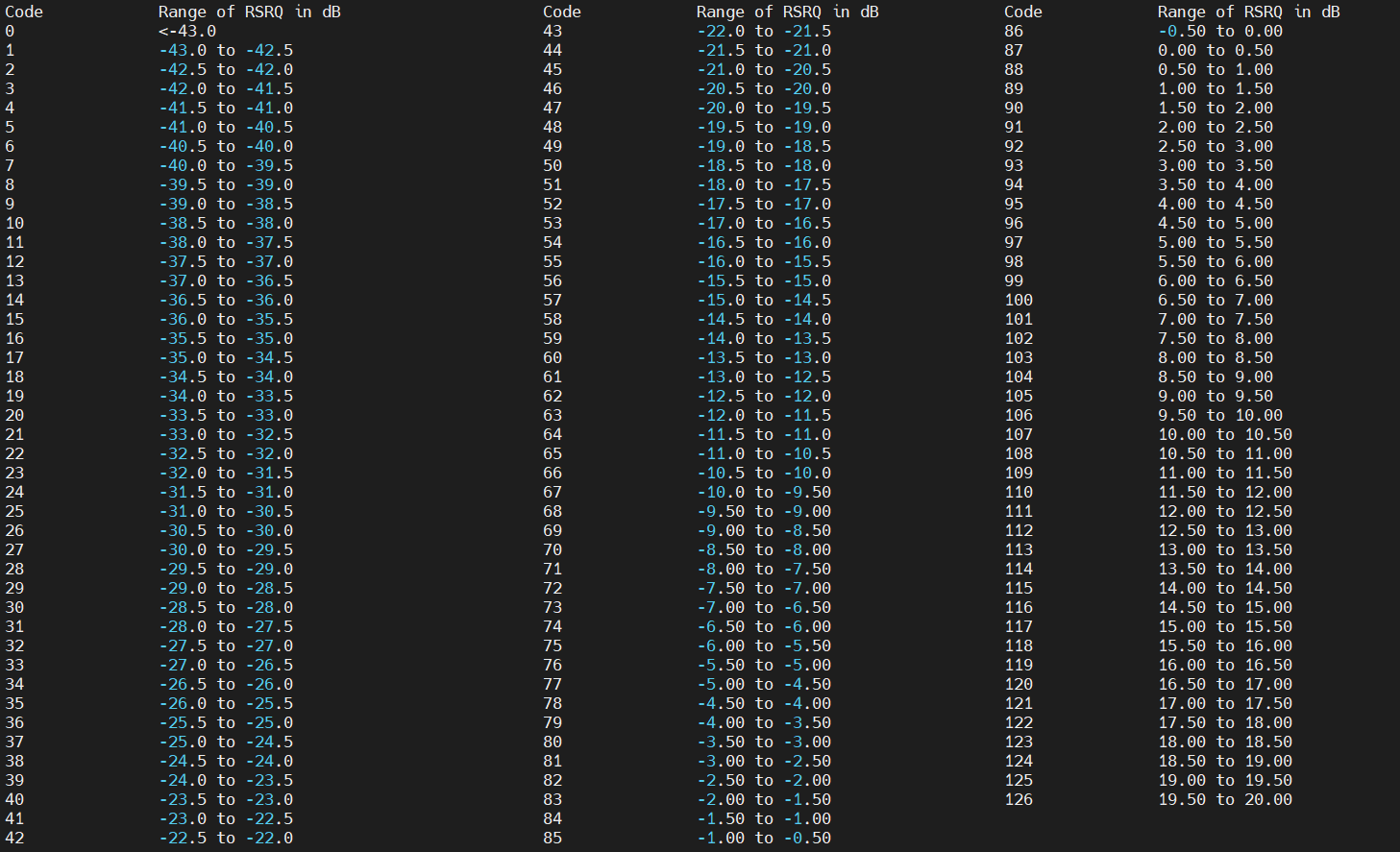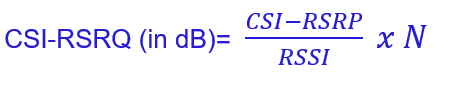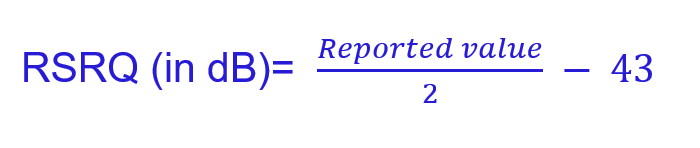# 5G NR RSRQ Measurements and Its Mapping

The RSRQ (Referece Signal RecivedQuality) is a measurement performed by the UE to know the Quality of recieved Reference Signals. In 5G NR, RSRQ can be measurement for SS-Reference Siganls and CSI-Reference Signals.

The SS-RSRQ measurements can be used for cell selection, cell reselection and mobility procedures where as CSI-RSRQ measurements can be used for mobility procedures only.

5G NR RSRQ Pointers

• It is the quantitive measure of recieved Reference Signals for SS (Sync Singal) or CSI ( Channel State Infonnation)
• RSRQ is measured in dB (decibal) where as RSSI and RSRP are measurement in dBm
• The RSRQ measurement can be used for cell selection, reselection and mobility (handover) procedures.
• There are two falvours of RSRQ namely SS-RSRQ and CSI-RSRQ
• 5G RSRQ range is defined as -43dB to 20 dB
• 5G RSRQ can be a positive or negative value where as LTE RSRQ is always a negative value
• The specification has defined a granularity of RSRQ as 0.5dB for measurement/represent
• UE does not report RSRQ is dB instead it reports an index value which is mapped to some RSRQ value by specification
• Full range of RSRQ (-43dB to 20dB ) with 0.5dB resolution can be mapped to 128 index value ( 7 bits) where Index #0 = -43 dB RSRQ and Index #127 = 20dB
• The Mapping for these Index value to absolute RSRQ value is provided within 3GPP Specification TS 38.133
• The mapping is common for SS-RSRQ and CSI-RSRQ
• UE reports RSRQ measurement to gNB within RRC: Measurement Report (MR) message, when gNB RRC configures Report Quantity for RSRQ
• 5G RSRQ measurements are filtered at Layer 3 to remove the impact of fast fading and to help reduce short term variations in the measurements

RSRQ Mapping with Indexes

Following table is shown Index Value and absolute RSRQ.RSRQ Theoretica Calculation

SS-RSRQ can be defined as the ratio of SS-RSRP and RSSI multiple by no. of Resource Block (RB) N.CSI-RSRQ can be defined as the ratio of CSI-RSRP and RSSI multiple by no. of Resource Block (RB) N.We can calculate the theoritical value for RSRQ using above formula considering following .

• RSRP range for 5G NR = -156 dBm to -31 dBm
• RSSI range for 5G NR = – 120 dBm to -13 dBm
• Resource Block for 5G (N) = 24 to 275 RBs

The above formulas for RSRQ calculation need to be coverted in logrithamic as RSRP (dBm) and RSSI (dBm) are log scale. In logritham the division (/) can represented as minus (-) and multiply with plus (+) and for RBs we need to covert them into 10 log (RBs).

• Minimum value for RSRQ = -156 – (-120) + 10*log(24) = -22.19 dB
• Index Value = 42
• Maximum Value for RSRQ= -31 – (-13) + 10*log (275) = 6.39 dB
• Index Value = 99

Calculated Practical RSRQ Value

Following formula can be used to calculate the RSRQ value in dB using the reported value by UE within measurement report.• UE Reported RSRQ Index Value = 45
• RSRQ (in dB) = 45/2 -43 = 22.5 – 43
• RSRQ (in dB) =  -20.5 dB

References:

• 3GPP TS 38.215 – 5G NR, Physical layer measurements
• 3GPP TS 38.133 – 5G NR, Requirements for support of radio resource management
• 3GPP TS 38.214 – 5G NR, Physical layer procedures for data

Related Post: